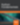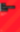# Back to Basics: Array Extras

JavaScript

Share:

#### Free JavaScript Book!Write powerful, clean and maintainable JavaScript.

RRP \$11.95

Arrays are a fundamental data structure in many programming languages, and JavaScript is no exception. To abstract away many of the details of working with arrays, JavaScript provides a collection of functions known as array extras. This article describes the various array extras and their uses.

## Background

Nearly all array operations are performed by looping over each array element, one at a time. For example, the following code uses a `for` loop to log all of the elements of an array to the debug console.

``````var foo = ["a", "b", "c", "d"];

for (var i = 0, len = foo.length; i < len; i++) {
console.log(foo[i]);
}``````

First, and foremost, you should understand that the previous example is perfectly good JavaScript code. However, if you have several complex loops, it can become tedious to keep track of variables. Array extras allow us to replace entire loops with function calls, often improving code readability. Now, let’s look at the various array extras.

## `forEach()`

Like many of the array extras, the `forEach()` method is a higher-order function – a function that receives another function as an argument. Instead of looping over array elements, `forEach()` invokes a callback function on every element in turn. The callback function accepts three arguments – the current array element, the array index, and the array itself. In the following code, the original example has been rewritten to use the `forEach()` method.

``````["a", "b", "c", "d"].forEach(function(element, index, array) {
console.log(element);
});``````

Notice that the use of `forEach()` eliminates the need for a loop and array subscript notation. Additionally, since JavaScript uses function level scoping, the `forEach()` callback function provides a new scope, allowing variable names to be reused. The one drawback is the performance hit that comes from invoking a function for each element in the array. Luckily, this penalty is often negligible. Also note that you can pass an optional argument to `forEach()` after the callback function. If present, this second argument defines the `this` value used within the callback function.

## `map()`

The `map()` function is nearly identical to `forEach()`. The only difference is that `map()` returns an array containing the values returned by the callback function. For example, the following code uses `map()` to compute the square root of each item in the input array. The results are then returned as an array and displayed. Also notice that the array extras are compatible with built in JavaScript functions, such as `Math.sqrt()`.

``````var sqrts = [1, 4, 9, 16, 25].map(Math.sqrt);

console.log(sqrts);
// displays "[1, 2, 3, 4, 5]"``````

## `filter()`

Like `forEach()` and `map()`, the `filter()` method takes a callback function and optional `this` value. And, like `map()`, `filter()` returns an array of values based on the return value of the callback function. The difference is that the `filter()` callback function should return a Boolean value. If the return value is true, then the array element is added to the results array. For example, the following code removes any elements from the original array that don’t begin with the letter x. In this example, a regular expression (passed as the `this` value) is tested against each array element.

``["x", "abc", "x1", "xyz"].filter(RegExp.prototype.test, /^x/);``

## `every()` and `some()`

The `every()` and `some()` functions also run a callback function on each array element. If every callback function returns `true`, then `every()` returns `true`, otherwise it returns `false`. Similarly, `some()` returns `true` if at least one callback function returns `true`. In the following example, `every()` and `some()` are used to test if array elements are less than five. In this case, `every()` returns `false` because the final element is equal to five. However, `some()` returns `true` because at least one element is less than five. Note that the `index` and `array` arguments exist, but have been omitted from the callback function because they are not needed in this example.

``````var foo = [1, 2, 3, 4, 5];
var every = foo.every(function(element) {
return element < 5;
});
var some = foo.some(function(element) {
return element < 5;
});
// every = false, some = true``````

## `reduce()` and `reduceRight()`

The `reduce()` method processes each element in an array, starting from the beginning, and computes a single value. `reduce()` takes a callback function and an optional initial value as arguments. If the initial value is not present, then the first array element is used. The `reduce()` callback function differs from the other callback functions we’ve seen thus far, as it takes four arguments – the previous value, current value, index, and the array.

A common example of a reduce operation is summing all of an array’s values. The following example does exactly this. The first time the callback function is invoked, `previous` is equal to one, and `current` is equal to two. In subsequent invocations, the sum is accumulated to a final value of 15.

``````var sum = [1, 2, 3, 4, 5].reduce(function(previous, current, index, array) {
return previous + current;
});
// sum = 15``````

The `reduceRight()` method works in the same fashion as `reduce()`, except that the processing begins at the end of the array and moves towards the beginning.

## `indexOf()` and `lastIndexOf()`

The `indexOf()` method searches an array for a specific element, and returns the index of the first match. If no match is found, `indexOf()` returns -1. `indexOf()` takes the element to search for as its first argument. A second, optional, argument is used to specify the starting index of the search. For example, the following code locates the first two occurrences of the letter z in an array. To find the second occurrence, we simply find the first occurrence, and then start searching again after it.

``````var foo = ["a", "z", "b", "z"];
var first = foo.indexOf("z");
var second = foo.indexOf("z", first + 1);
// first = 1, second = 3``````

The `lastIndexOf()` method works exactly the same way, except it starts searching from the end of the array.

## Conclusion

Utilizing the array extras can lead to clean, concise code. Sadly, some older browsers do not support these methods. However, you can detect these methods by inspecting the `Array.prototype` object (i.e. `Array.prototype.forEach`). If a method is missing, you can easily provide your own implementation.### Colin Ihrig

Colin Ihrig is a software engineer working primarily with Node.js. Colin is the author of Pro Node.js for Developers, and co-author of Full Stack JavaScript Development with MEAN. Colin is a member of the Node.js Technical Steering Committee, and a hapi core team member. Colin received his Bachelor of Science in Engineering, and Master of Science in Computer Engineering from the University of Pittsburgh in 2005 and 2008, respectively.

#### New books out now!Learn valuable skills with a practical introduction to Python programming!Give yourself more options and write higher quality CSS with CSS Optimization Basics.